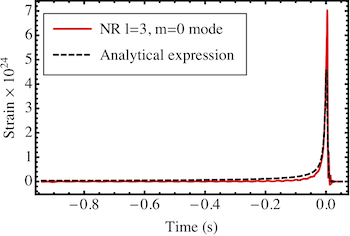# Research Theme: Extended BMS Algebra, Spin and Center-of-Mass Memory Effects

This post summarizes three papers related to the conserved quantities of an extension of the Bondi-Metzner-Sachs (BMS) group and two related new memory effects.

## Summary of the PapersThe Bondi-Metzner-Sachs (BMS) group is the symmetry group of asymptotically flat spacetimes, and it consists of the Lorentz transformations and the supertranslations (an infinite-dimensional commutative group that has the spacetime translations as a subgroup). There recently was a proposal that a larger symmetry algebra than the BMS algebra could be relevant. This new algebra was called the extended BMS algebra, and it included the infinite number of conformal Killing vectors on the two-sphere, not just the smooth six-parameter family of Lorentz transformations. We computed the conserved quantities corresponding to these new symmetries in a somewhat more general context than before, and we found that they are finite and that they have an electric- and a magnetic-parity part. These two parts are generalizations of the spin angular momentum and the center-of-mass parts of the relativistic angular momentum (the superspin and super-center-of-mass charges, respectively). The change in the superspin charges can be a source of a new kind of memory effect, which had been proposed earlier, called the spin memory effect.

Another source of the spin memory effect is the effective angular momentum per solid angle that is radiated in gravitational waves. I recently computed the expansion of this effective angular momentum flux in terms of radiative moments of the gravitational-wave strain, thereby deriving a quadrupole-like formula for the spin memory. I then specialized to compact binary sources in the post-Newtonian approximation. In this limit, I found that the spin memory has a substantial slow secular growth during the inspiral stage of a compact binary. In addition, the rate of accumulation of the spin memory is related to a non-hereditary, nonlinear, and non-oscillatory contribution to the gravitational waveform that appears at a high order in the post-Newtonian expansion. This rate of accumulation of the spin memory might be detectable by third-generation gravitational-wave detectors, such as the Einstein Telescope, by coherently adding the spin memory signals from hundreds of gravitational-wave observations of black-hole-binary mergers.

I also showed that changes in the super-center-of-mass charges can also produce a new type of memory effect, which I called the center-of-mass memory effect. Like the spin memory effect, it produces a lasting change in a quantity related to the time integral of the gravitational-wave strain. There are terms in the gravitational waves related to the center-of-mass memory effect. These gravitational-wave terms, however, will be weaker than those related to the spin memory effect. As a result, it is less likely that the next generation of gravitational-wave detectors will be able to find evidence for the effect. It should, however, appear in the post-Newtonian expansion of the gravitational waveform, when the gravtiational waveform is computed at fourth post-Newtonian order.

Categories:

Updated: